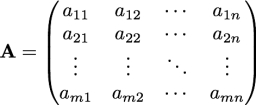# The sum 17

The sum of the three numbers is -1. If we multiply the second number by 2, the third number by 3, and add them, we get 5. If we subtract the third number from the sum of the first and second numbers, we get -1.

Represent the above information algebraically. Convert the system of linear equations into matrix form. If the matrix formed by coefficients of variables is written by A, then find |A|. Find adj (A), where the adjoint of A is the transpose of the matrix of the co-factors. Find the numbers using the matrix method.

a =  -7
b =  6
c =  0

### Step-by-step explanation:

a+b+c=-1
a+2b+3c=5
a+b-c=-1

a+b+c=-1
a+2·b+3·c=5
a+b-c=-1

a+b+c = -1
a+2b+3c = 5
a+b-c = -1

Row 2 - Row 1 → Row 2
a+b+c = -1
b+2c = 6
a+b-c = -1

Row 3 - Row 1 → Row 3
a+b+c = -1
b+2c = 6
-2c = 0

c = 0/-2 = -0
b = 6-2c = 6 = 6
a = -1-b-c = -1-6 = -7

a = -7
b = 6
c = -0

Our linear equations calculator calculates it.Did you find an error or inaccuracy? Feel free to write us. Thank you!

Tips for related online calculators
Do you have a system of equations and looking for calculator system of linear equations?